Technical Report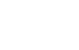What is dB?
(page2)

4.Decibels in various fields

Here, we will concretely describe how decibels are used in various fields.

4-1 Electrical/communication system

Decibels of the relative level value are widely used for the gain and attenuation of the transmission system. Since the normal voltage measurement is easier, calculated the decibel by taking the voltage ratio in the calculation of the transfer characteristics including amplifiers or filters.

For example, if a signal of 1 mV is input to a circuit, a voltage of 10 V is generated at that output. In this case, since the voltage amplification factor is 10,000 times, "gain is 80 dB" using equation (3-4).

Below the decibels that are often used in electrical and communication systems are introduced.

dBm
In the field of power amplification, dBm is often used . This unit is the absolute level value of electric power, and the value of 1 mW is defined as 0 dBm. For example, how 10 W is expressed in dBm? In this case, since 10 W is equal to 104 mW, it is 40 dBm(= 10 log (10 4) ).
In the same way, how 0.1 mW is expressed in dBm? In this case, since 0.1 mW is 10-1 mW, it is -10 dBm.

Although dBm is originally a unit of electric power, in the transmission system, it is also used as the absolute level value of voltage by fixing the impedance.
Assuming now that the voltage value of 0 dBm (1 mW) is V when the impedance system is Z (Ω), the relation of the expression is obtained as follows.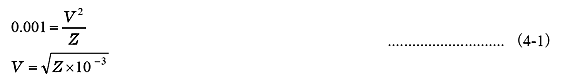The Table 6 shows examples of voltage values for 0 dBm at various impedance values from equation (4-1).

 Table.6　Voltabe value for 0 dBm
 Impedance Z (Ω) Voltage value (V) The field often used 50 0.224 Wireless system 75 0.274 Video system 600 0.775 Phone acoustic system

A correlation formula between the voltage value V and the decibel value X(dBm)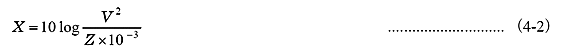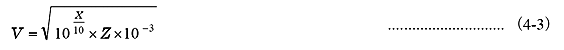(Example 1) 0.5 V is 7.0 dBm when 50 Ω
(Example 2) 1 V is 11.2 dBm when 75 Ω
(Example 3) 6 dBm is 1.55 V when 600 Ω

dBV,dBμ
These are the absolute level value of electric power, and the value of 1V is defined as 0 dBV, and 1 μV is defined as 0 dBμ.

Note

Basically, the voltage value to be leveled (antilogarithm) will be the effective value of the signal.

dBV is often used for low-frequency band instruments such as FFT analyzer. In the FFT analyzer, the unit of input is voltage (V) by default, so if calibration of the physical quantity is not made, the vertical axis unit of power spectrum is [dBV] notation.

Also, the input voltage range is usually 10 dBV steps. For example, for the input range of the DS-3000 series Data Station, voltage 1 Vrms will be 0 dBV voltage (see Table 7).

 Table7.　Input range table of the DS-3000 series
 dBV Vrms V(peak) 20 10 ±14.14 10 3.162 ±4.471 0 1 ±1.414 -10 0.3162 ±0.4471 -20 0.1 ±0.1414 -30 31.62 m ±44.71 m -40 10 m ±14.14 m

A correlation equation between the voltage value V and the decibel value X(dBV)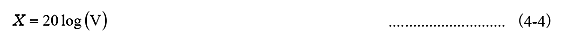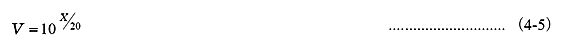dBμ is often used for wireless communication and is based on the voltage value of 1 μV. Although originally it should be expressed as dBμV, V is often omitted.

dBμV/m
This unit is used to represent the electric field intensity value expressing the radiation emission value, such as EMC. 1 μV/m is defined as
0 dB.

dBc
This is a relative level value of harmonic wave based on the fundamental wave ("c" of Carrier) or noise components , which is used when evaluating the spectral characteristics of high frequencies and OP amplifier.
For example, when harmonic wave is -40 dBm which fundamental wave is 10 dBm, its harmonic wave is -50 dBc. This relationship is the same even if the vertical axis of the spectrum represents dBV. It is used as a parameter to evaluate low distortion such as OP amplifier.

dBV/√Hz
When measuring the self-noise characteristics of an amplifier, the power spectral density (PSD) is used for evaluation, but since this vertical axis is an effective value per unit frequency (1 Hz), the unit is V2/Hz or V/√Hz. This value is expressed in decibels as dBV/√Hz.

4-2 Acoustic system

To quantify the strength (magnitude) of sound, decibel (dB) is also commonly used. The major reason is that we can feel very wide range of sound strength (magnitude) and that feeling is logarithmic (see (3) in 3.4) . Quantification of volume is often expressed in absolute level value of decibel (dB).

Sound pressure level [Lp]
Sound traveling in the air is a minute pressure fluctuation (wave) centered on atmospheric pressure (static pressure). The effective value of the fluctuating component is called sound pressure, and Pa (Pascal) is that unit. As mentioned above, the sound pressure that human beings usually can hear is ranging from 20 μPa to 20 Pa, very wide range of value 106.

The sound pressure level is defined by the following formula.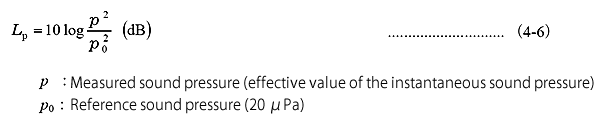Note

The sound pressure level is the strength (magnitude) of the physical sound. In the sound measurement field, the level with frequency weighting A, which is decided based on human auditory characteristics, is often used, and in particular, it is called A-weighting sound pressure level (commonly the noise level) . It is defined by the following expression.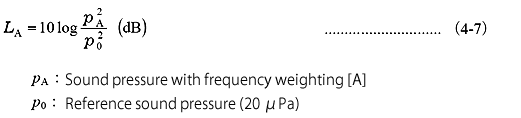In equations (4-6) and (4-7), how to specifically obtain the sound pressure (the effective value of the instantaneous sound pressure) is a question. Normally, the following average method is often used to find the effective value from the instantaneous sound pressure waveform.

Exponential average

In this method, the square value of the instantaneous sound pressure is exponentially averaged with a certain time weight τ, and the effective value at that time (instantaneous) is obtained. The decibel value obtained by this method is also a function of time. The so-called instantaneous sound pressure level corresponds to this, and it is usually displayed every 1 second on the sound level meter. In JIS standard (JISC 1509) of the sound level meter, it is called sound level with time weighting. In the field of acoustic measurement, Fast (0.125 s) and Slow (1 s) are often used for time weighting τ (time constant).

Linear average

In this method, the square value of the instantaneous sound pressure is integrated (by equal weighting) at a certain measurement time T and then averaged to obtain the effective value. The decibel value to be obtained from this is the representative value at that measurement time (one value). In JIS standard (JIS C1509) of the sound level meter, it is called time average sound level (equivalent sound level). When the frequency weighting is [A], it becomes "equivalent noise level" in the environmental noise field. For details, please refer to "Chapter 9 Sound Level Meter Readings" in "What is sound level meter?"of Ono Sokki web site .

Sound strength (sound intensity) level [LI]
Sound intensity is the energy that passes through a unit area of sound waves that travel in a medium such as air within a unit time.
The unit is [W/m2].

Sound intensity level is defined by the following expression.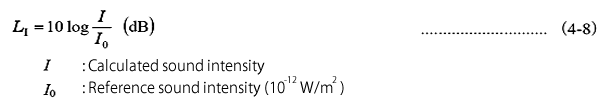When a sound wave can be regarded as a plane wave, the relationship between sound intensity I and sound pressure p is: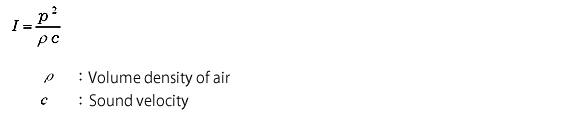As the relationship shown above, the sound intensity level L1 is almost equal to the sound pressure level Lp. On the correspondence between sound intensity and sound pressure, please refer to Ono Sokki website, Figure 5.1 of "5-1 sound pressure level" in "What is sound level meter?" .

Sound power level [LW]
A sound wave propagating in a medium can be thought of as a flow of energy, and this energy is called an acoustic energy. As an amount representing the magnitude of this acoustic energy, the acoustic energy passing through a specified plane in unit time is called "sound power"[P(W)].

Sound power level is defined by the following expression.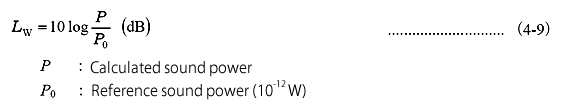The sound power is mainly used to express the magnitude of the acoustic energy radiated from the sound source.
Within a specified frequency band, the total acoustic energy radiated by the sound source per unit time is called "sound output (sound power of the sound source)"[P(W)]and its sound power level is called "sound output level (sound power level of the sound source ) "[LW](dB).

Acoustic energy level [LJ]
For the sound sustainably generated, the above sound power level is used as an evaluation method. For one-shot or transient sound, it is necessary to evaluate with energy.

The acoustic energy level [LJ] is defined by the following equation.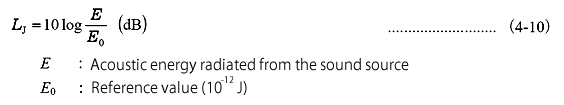4-3 Vibration system

Absolute decibel values are often used in the field of vibration system, especially in the field of human body vibration, like the acoustic system.

Vibration acceleration level [LVa]
Usually, the acceleration signal is used as the measurement amount of vibration.
Actual vibration is not a simple waveform like a sinusoidal wave but a complicated signal including various frequency components, so its magnitude is expressed using effective values that correspond to energy and power.

Vibration acceleration level [LVa] is defined by the following formula.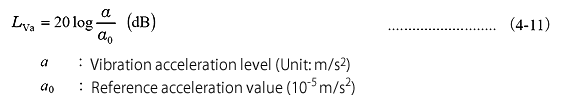The reference acceleration value is 10-5 m/s2 in JIS, but 10-6 m/s2 is the reference in ISO. That is, difference of 20 dB in the vibration acceleration level.

 [e.g.] For example, practical unit "Gal" is used in the seismic field. 1 Gal is 1 cm/s2, so 1 Gal corresponds to 60 dB for JIS and 80 dB for ISO.

 Note Energy is integral of power. As a unit, it has the dimension of (power) × (time). Conversely, power corresponds to energy per unit time. For example, in the field of electricity, electricity is power and its unit is kW, the amount of electricity is energy and its unit is kWh.

Vibration level [LV]
The vibration level is the level value of the effective value of the vibration acceleration which made correction so as to make it close to the human sense of vibration. The influence of vibration on the human body depends on the amplitude and frequency, and how the vibration feels differs in the vertical direction and the horizontal direction. This is stipulated in JIS C 1510-1995 as "Overall frequency response of vibration sensory characteristics".

The vibration level [LV] is defined by the following formula.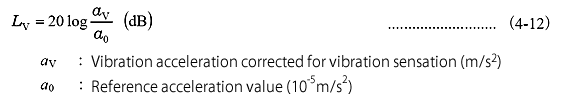The vibration level is a decibel value equivalent to the A weighting sound pressure level (noise level) in the sound system.

Note

Same as the acoustic system, the effective value is obtained by using the averaging method, as shown below.

• Exponential average
In this method, the square value of the instantaneous sound pressure is exponentially averaged with a certain time weight τ, and the effective value at that time (instantaneous) is obtained. The decibel value obtained by this method is also a function of time. The so-called instantaneous sound pressure level corresponds to this, and it is usually displayed every 1 second by vibration level meter.
In JIS standard (JIS C 1510-1995) of the vibration level meter, time weighting τ (time constant, dynamic characteristics) is
0.63 s.

• Linear average
In this method, the square value of the instantaneous sound pressure is integrated (by equal weighting) at a certain measurement time T and then averaged to obtain the effective value. The decibel value to be obtained from this is the representative value at that measurement time (one value). This value is called Equivalent vibration level ( LVeq ), as the same meaning for the sound system.